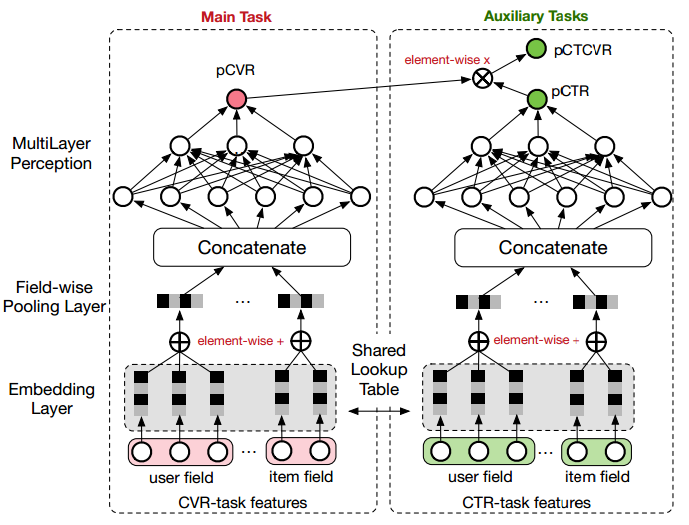ESMM模型有两个主要的特点:

• 在整个样本空间建模。区别与传统的CVR预估方法通常使用“点击->成交”事情的日志来构建训练样本，ESMM模型使用“展现->点击->成交”事情的日志来构建训练样本。
• 共享特征表示。两个子任务（CTR预估和CVR预估）之间共享各类实体（产品、品牌、类目、商家等）ID的embedding向量表示。

ESMM模型的损失函数由两部分组成，对应于pCTR 和pCTCVR 两个子任务，其形式如下：
\begin{align}
L(\theta_{cvr},\theta_{ctr}) &=\sum_{i=1}^N l(y_i, f(x_i; \theta_{ctr}))\\
&= \sum_{i=1}^N l(y_i\&z_i, f(x_i; \theta_{ctr}) \times f(x_i; \theta_{cvr}))
\end{align}

ESMM模型由两个结构完全相同的子网络连接而成，我们把子网络对应的模型称之为Base模型。接下来，我们先介绍下如何用tensorflow实现Base模型。

## Base模型的实现

### 模型函数

Base模型的其他组件就不过多介绍了，模型函数的代码如下：

## ESMM模型的实现

• For mode == ModeKeys.TRAIN: required fields are loss and train_op.
• For mode == ModeKeys.EVAL: required field is loss.
• For mode == ModeKeys.PREDICT: required fields are predictions.